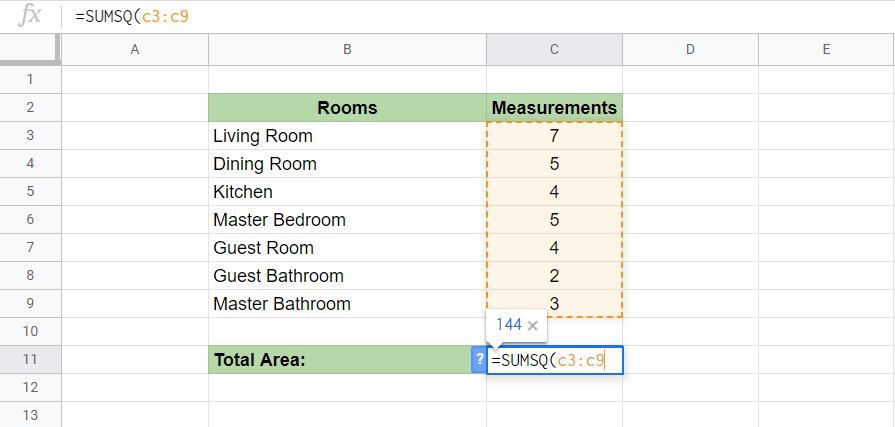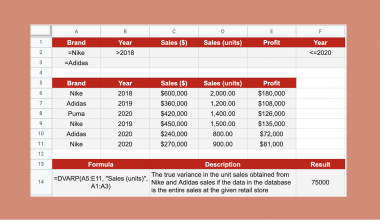# How to Use SUMSQ Function in Google Sheets (Sum of Squares)

The SUMSQ function is a Google Sheets command that is used to return a sum of a series of squares.

The SUMSQ function is a relatively simple function that combines two operations, looking for the sum and squaring a series of numbers, into one command.

Instead of manually making a table of PRODUCT functions to look for the square, and another cell to compute the SUM, by using SUMSQ you’ll be able to simplify the process.

Here’s a quick example.

As a test exercise for architectural or engineering students, you have to calculate the entire floor space of multiple square rooms. You’ll need the measurements of each room, and since they’re square, measuring one wall length is enough. To find the area of one room, you will need to multiply the length and the width of the room.

Simple, since the rooms are square, the length and width will be the same. Just make a data table with all your encoded measurements for every room and have these values as the reference cells of the SUMSQ function.

We’ll expound this further on with a more detailed example, after going over the specific components of the SUMSQ function.

## The Anatomy of the SUMSQ Function.

The syntax (the way we write) of SUMSQ functions is pretty simple.

```=SUMSQ(A2:A10)
=SUMSQ(5, 7, 9, 11, 13)
=SUMSQ(5, 7, A5:A10)```

Let’s breakdown what each term means and understand the three ways we can implement this function.

• SUMSQ is the function that sums the square of a set of numbers.
• A2:A10 is the set of numbers from cells A2 and A10.
• 5, 7, 9, 11, 13  are manually inputted numbers in the function, unlike cell references such as A2.
• 5, 7, A5:A10 is a collection of manually inputted numbers as well as a set of numbers from cells A5 to A10.

With the SUMSQ function, you won’t have to make two tables of the PRODUCT function and the SUM function to get what you want. Additionally, since the SUMSQ automatically squares a number, this will save you time in contrast to the PRODUCT function where you will have to manually multiply the number to itself.

Furthermore, the SUMSQ function allows you to have complete control with the reference cells that you’re operating on. By being able to isolate (A2, A3, A4) and group together (A2:A4) cell blocks, you can specify your reference cells with ease.

## A Real Example of Using the SUMSQ Function.

Let’s look at this example below to see how we can apply the SUMSQ function in Google Sheets.

### Find the Area of 5 Square Rooms in Google Sheets

In this simple problem, we’ll be computing the total area of 5 square rooms of different sizes.The function with a cell reference is:

`=SUMSQ(B3:B7)&" ft"`

As a result, we will get 479ft as the total area of the 5 rooms.

You can try this simple problem yourself! Click the link below to make a copy of our spreadsheet sample:

## How To Use The SUMSQ Function in Google Sheets.

In this section, we’ll walk you through a step-by-step guide on how to use the SUMSQ function in Google Sheets.

### Finding the Total Area of A House With 7 Square Rooms in Google Sheets

1. Let’s start by encoding the sheets with the necessary data. After that, we’ll be choosing the cell that will display the total area. For this guide, we’ll use C11. Click the cell to make it active.1. Now, type in ‘=’ to start off the function. Complete the function by typing ‘sumsq‘ or ‘SUMSQ’. Feel free to auto-complete the function if you don’t feel like typing the entire thing.1. Then, start accepting parameters by typing in ‘(‘. After that, input the beginning cell reference. In this example, it’s C3.1. Now finish the SUMSQ function by grouping the cells together with ‘:’ as a connector to the last reference cell, ‘C9’.1. Quickly finish the function with the closing bracket ‘)’.1. Since we’re measuring a house, we’ll have to display the area with the proper unit. After the ‘)’ add in a ‘&’ so we can add the last bit of detail.1. Go ahead and type in ‘“m”’ to specify that the total area is in meters.1. Finally, press Enter and watch the function display the total area of the house in meters! Each room should now be squared and summed together to display the total area.That should be all you need! You can now use the SUMSQ function together with other Google Sheets formulas and create even more creative and amazing formulas.

##### You May Also Like## How to Use TODAY Function in Google Sheets

The TODAY function in Google Sheets is useful to return the current date. We can use the TODAY…## How to Use the IMCOT Function in Google Sheets

The IMCOT function in Google Sheets returns the cotangent or arctangent of a complex number. The cotangent of…## How to Create a Dependent Drop Down List in Google Sheets

Learning how to create a dependent drop-down list in Google Sheets is useful to create several lists of…## How to Use DVARP Function in Google Sheets

The DVARP function in Google Sheets is useful if you need the variance of a population, based on…